# The time sequence for a flat-field beam

The time sequence for a collimated flat-field beam laser pulse entering a nonscattering absorbing medium at an air/medium interface.

Absorption coefficient μa = 50 cm-1 = 5000 m-1.
Irradiance E = 0.0200 [J/cm2] = 200 [J/m2].
Energy deposition W = μaE = 1 [J/cm3] = 106 [J/m3].
Peak pressure = ΓW =(0.12)(106 [J/m3]) = 1.2x105 [J/m3] = 1.2x105 [Pa].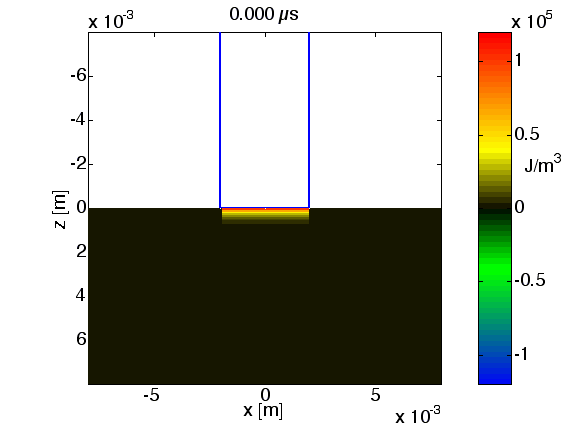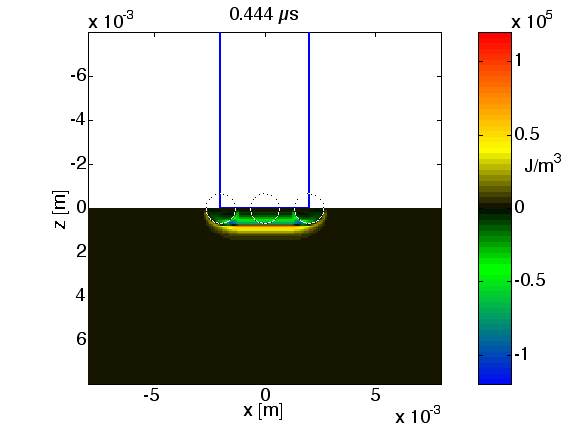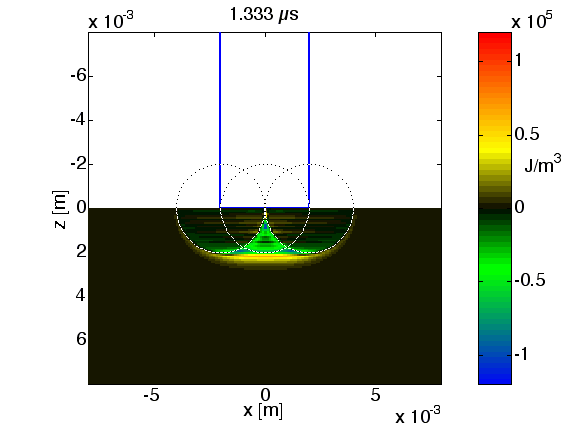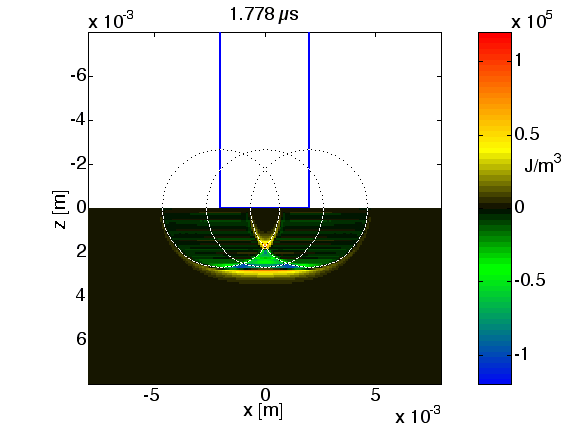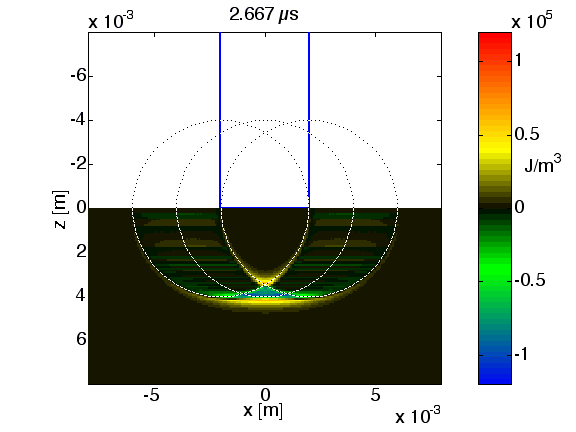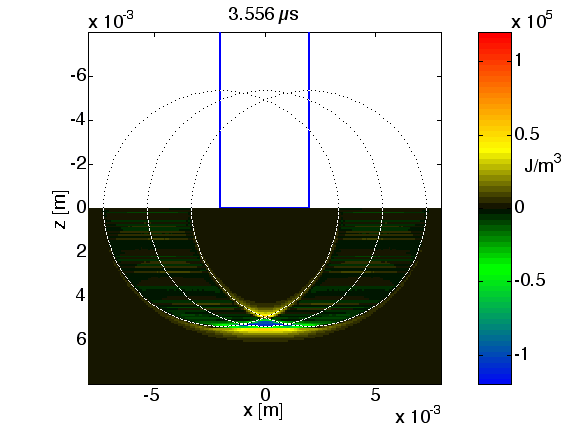The key features are the leading edges of positive compressive pressure (red) and negative tensile pressure (blue). The region of energy deposition under the beam yields a strong positive pressure (red). The edge of the circular laser beam elicits negative pressure that concentrates near the central axis (strong blue) as the pressure wave moves into the tissue. Reflections from the tissue-air interface cause this edge effect to elicit a final positive pressure along the central axis.

This early computation still suffers from some computational noise in the low pressure regions, so do not attach too much significance to pressures fluctuating close to zero (slight green or yellow). Work in progress :-)